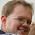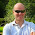## Tuesday, May 13, 2014

### Propnet basics

I want to spend a few posts talking about our implementation of propnets (propositional networks), which are a representation of a game state machine in terms of:

• A set of propositions, which may be true or false in any given state, and which together make up that state.  These are known as the 'base propositions'.  An example might be 'cell (2,2) contains an X' in TicTacToe.
• Some informational (output) propositions, such as 'this state is terminal', or 'this state scores 75 for player 1'
• (Ouput) Propositions corresponding to every possible move in the game, which are true when that move is legal in the current state
• (Input) Propositions corresponding to every possible move in the game, set true when the associated move is being played (these will be in (1-1) correspondence with the legal propositions)
• Outputs (known as transitions) corresponding to each base proposition, whose value is the value that the corresponding base proposition would take (in the successor state to the current state), if the moves indicated by the input move propositions were played
Each of the above, and all the connective logic is represented by logical 'components' in what amount to a virtual logic circuit.  The connective logic is formed of components implementing the standard logical operators (AND, OR, NOT) plus input-less components representing Boolean constants.

When implementing propnets you have two major choices to make, which broadly categorize the different types of implementation:
1. How to perform propagation of the input values through the network efficiently.  The major choices are forward propagation (set the inputs, then calculate the components they immediately connect to, then the ones those connect to, and so on), and backward propagation (query the output proposition you're interested in and determine its state by recursively querying its inputs, and so on)
2. How to represent the current state of the network.  Here I will split the discussion between what I am going to call internal representations and external representations.  In an internal representation the components that form the network hold their own state internally.  In an external representation the components themselves are stateless and some external structure holds their current state, so the components simply describe the network topology and semantics, not its current state.
The initial version of Sancho used a differentially-forward propagated internally represented approach.  This has changed recently, with significant performance gains resulting from a change to an external representation, which I'll be talking about in detail in a future post.  In the remainder of this post I will describe the propagation mechanism, and then in the next post I will discuss a number of optimizations.  Finally, in the post after that, I will discuss the move to an external representation and the benefits that brought with it.

Forward Propagation

At its simplest, forward propagation works (approximately) as follows:

The input values to the network are set, and each processed in turn by signalling to components connected immediately to the inputs that they should recalculate.  On being told to recalculate a component will:
1. Query its input values and from them calculate its new output value
2. Signal to any components connected to its output that they should recalculate
This causes recursion through the network, ultimately resulting in the new state of (all) outputs being calculated.  It can be thought of as a very direct modelling of an actual circuit (and actually implementing the logic as a physical circuit, say with a programmable logic array is certainly an interesting possibility).

As it stands this is not very efficient, because the same components recalculate their state many times over (each time an input is recalculated to any given component, and that may be one of many).  A standard way to resolve that is to pre-calculate a dependency ordering for the network, and recalculate component states in that order (so an output is not calculated before all of its inputs, and thus only needs to be calculated once), which is loosely analogous to imposing a clock on logic circuitry. If loops occur this needs a little refinement (loops are actually very rare and only occur in certain types of game description), but even used naively is generally reasonably effective.

However, we do not calculate any dependency ordering, and our solution for this first problem drops out of that for the next, which is that even if you calculate a dependency ordering and thus calculate each component just once for each state transition, there is still a major inefficiency, which arises from the typical usage patterns.

When playing most games the state evolves from turn to turn only by small changes (e.g. - one piece moves from one position to another, or a cell is marked on a board and so on).  As a result most of the base propositions remain unchanged across the vast majority of state transitions.  This means that most of the components in the propnet will have the same inputs as they did on the previous calculation, and if we can avoid recalculating them, then we should make major gains.

Differential Propagation

We can easily achieve this by holding two values in each component - the current state and the last propagated state for that component's output.  With that tweak we can modify the recalculate behavior of the component to:
1. Query its input values and from them calculate its new output value
2. Compare the new output value with the last propagated output value.  Only if they differ, signal to connected components that they need to recalculate
This also (largely) addresses the first problem and makes pre-calculation of the dependency ordering unnecessary.  It still has some residual benefit, because even with differential propagation more than one input to any given component could still change (causing it to be signaled multiple times).  However, this turns out to be almost a non-issue with one further change, which is itself a significant overall optimization.  In many ways the discussion of this optimization belongs in the planned next post, but because of the synergy with the propagation mechanism I'll describe it here.

Optimizing input querying

With the basic implementation above, it turns out that a major cost is in each signaled component querying its inputs.  In many generated networks some components will have a large number of inputs (many tens of inputs is very typical).  Simply iterating over those inputs and retrieving their values becomes a major cost.  However this can be entirely avoided if we leverage the fact that we have our previous state held in order to perform differential propagation!

First note that the only components that can have multiple inputs are AND and OR components.  Given that, suppose we not only hold the current output state in each component but also a count of the number of inputs whose value is true.  Given a component with N inputs we'll call the number of true inputs T say.  For an AND component the output is (N==T), and for an OR it is (T!=0).  If components only signal their outputs to recalculate when their output state actually changes it follows that being signaled with true implies T := T+1, and with false that T := T-1.  Thus we can dead-reckon the new output state without having to query any other input at all.

This is not only much more efficient than looping over all the inputs, but also resolves the remaining 'multiply calculated' issue fairly effectively (most input changes won't change the output state, and empirically there isn't much left to be gained by doing things in dependency order)

In my next post I'll discuss a wide range of optimizations, both small scale and large.

1.You mention near the end about "...also resolves the remaining 'multiply calculated' issue ...". How do you handle the case where the value might flipflop depending on the changing value of the inputs which may cause extra unnecessary propagation. eg. a 2-input OR gate with 1 input initially true and then both inputs flip (in an undetermined order). Both changes will cause a propagation if handled independently. Whereas they wouldn't cause any propagation if handled together (or with a dependency ordering)

2.We don't bother doing anything special to handle that case. We could, but it isn't worth it. That's what Steve meant by "empirically there isn't much left to be gained by doing things in dependency order".

3.As Andrew says. Experimentally it turned out that the cost of policing it outweighed the cost of the residual cases occurring. Typically this flip-flopping doesn't actually happen much, and when it does it tends to die off at the next component the flip-flopping component feeds into. I think this is probably because components with a fairly large number of inputs turn out to be rather common, and these have a strong tendency to suppress this behavior.
Having said that, one usage optimization we make is to further minimize a somewhat common case where it does occur, which is when calculating a next state you have to set, and afterwards reset, a 'does' proposition for the move you are examining. We found that not bothering to do the reset until after you've set the NEXT move proposition (for the NEXT move you want to examine) reduces overall propagation time, and it is because it removes some of this flip-flopping. To achieve this we cache the 'last set move prop', and when querying during getNextState() reset the cached move prop only after we set the newly queried one (then updating the cache to reference that one of course)

4.Love the last trick, and it opens the road to further optimizations. Hope you don't mind if I use it, should be easy to add it to my QFWFQ player as it already does differential propagation.

1.Go right ahead. Th whole point of this blog is to encourage an exchange of techniques, which ultimately benefits us all.